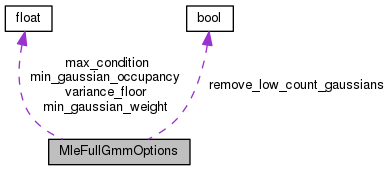MleFullGmmOptions Struct Reference

Configuration variables like variance floor, minimum occupancy, etc. More...

`#include <mle-full-gmm.h>`

Collaboration diagram for MleFullGmmOptions:[legend]

## Public Member Functions

MleFullGmmOptions ()

void Register (OptionsItf *opts)

## Public Attributes

BaseFloat min_gaussian_weight
Minimum weight below which a Gaussian is removed. More...

BaseFloat min_gaussian_occupancy
Minimum occupancy count below which a Gaussian is removed. More...

BaseFloat variance_floor
Floor on eigenvalues of covariance matrices. More...

BaseFloat max_condition
Maximum condition number of covariance matrices (apply floor to eigenvalues if they pass this). More...

bool remove_low_count_gaussians

## Detailed Description

Configuration variables like variance floor, minimum occupancy, etc.

needed in the estimation process.

Definition at line 38 of file mle-full-gmm.h.

## ◆ MleFullGmmOptions()

 MleFullGmmOptions ( )
inline

Definition at line 49 of file mle-full-gmm.h.

49  {
50  min_gaussian_weight = 1.0e-05;
51  min_gaussian_occupancy = 100.0;
52  variance_floor = 0.001;
53  max_condition = 1.0e+04;
55  }
BaseFloat variance_floor
Floor on eigenvalues of covariance matrices.
Definition: mle-full-gmm.h:44
BaseFloat min_gaussian_weight
Minimum weight below which a Gaussian is removed.
Definition: mle-full-gmm.h:40
BaseFloat min_gaussian_occupancy
Minimum occupancy count below which a Gaussian is removed.
Definition: mle-full-gmm.h:42
BaseFloat max_condition
Maximum condition number of covariance matrices (apply floor to eigenvalues if they pass this)...
Definition: mle-full-gmm.h:47

## ◆ Register()

 void Register ( OptionsItf * opts )
inline

Definition at line 56 of file mle-full-gmm.h.

References OptionsItf::Register().

Referenced by main().

56  {
57  std::string module = "MleFullGmmOptions: ";
58  opts->Register("min-gaussian-weight", &min_gaussian_weight,
59  module+"Min Gaussian weight before we remove it.");
60  opts->Register("min-gaussian-occupancy", &min_gaussian_occupancy,
61  module+"Minimum count before we remove a Gaussian.");
62  opts->Register("variance-floor", &variance_floor,
63  module+"Minimum eigenvalue of covariance matrix.");
64  opts->Register("max-condition", &max_condition,
65  module+"Maximum condition number of covariance matrix (use it to floor).");
66  opts->Register("remove-low-count-gaussians", &remove_low_count_gaussians,
67  module+"If true, remove Gaussians that fall below the floors.");
68  }
BaseFloat variance_floor
Floor on eigenvalues of covariance matrices.
Definition: mle-full-gmm.h:44
BaseFloat min_gaussian_weight
Minimum weight below which a Gaussian is removed.
Definition: mle-full-gmm.h:40
BaseFloat min_gaussian_occupancy
Minimum occupancy count below which a Gaussian is removed.
Definition: mle-full-gmm.h:42
BaseFloat max_condition
Maximum condition number of covariance matrices (apply floor to eigenvalues if they pass this)...
Definition: mle-full-gmm.h:47

## ◆ max_condition

 BaseFloat max_condition

Maximum condition number of covariance matrices (apply floor to eigenvalues if they pass this).

Definition at line 47 of file mle-full-gmm.h.

Referenced by main(), and kaldi::MleFullGmmUpdate().

## ◆ min_gaussian_occupancy

 BaseFloat min_gaussian_occupancy

Minimum occupancy count below which a Gaussian is removed.

Definition at line 42 of file mle-full-gmm.h.

Referenced by kaldi::MleFullGmmUpdate().

## ◆ min_gaussian_weight

 BaseFloat min_gaussian_weight

Minimum weight below which a Gaussian is removed.

Definition at line 40 of file mle-full-gmm.h.

Referenced by kaldi::MleFullGmmUpdate().

## ◆ remove_low_count_gaussians

 bool remove_low_count_gaussians

Definition at line 48 of file mle-full-gmm.h.

Referenced by kaldi::MleFullGmmUpdate().

## ◆ variance_floor

 BaseFloat variance_floor

Floor on eigenvalues of covariance matrices.

Definition at line 44 of file mle-full-gmm.h.

Referenced by main(), and kaldi::MleFullGmmUpdate().

The documentation for this struct was generated from the following file: